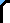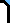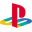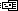Cheats DS GBA PC PS2 PS3 PSP Wii Xbox Xbox 360 New ReviewsSite Search

This Site WebWarhawkEmail this Page  |Printer Friendly Version

```Level Passwords & Special Passwords.

Passwords: Enter these codes at the "Special Access" (password) screen:

Level Passwords:
Desert Level: Triangle Square X Triangle X X Square Square
Canyon Level: Circle Triangle Triangle X Circle Circle Square Circle
Airship Level: X Triangle X Square Square Triangle Square Triangle
Volcano Level: Square Triangle X X X Triangle Circle Square
Gauntlet Level: Triangle Circle Triangle Circle Triangle X Square Square
Stormland: Triangle X Triangle Square Circle X Circle Circle

Special Passwords:
Warhawk A-La Mode (infinite weapons and invincibility): Circle, Circle,
Circle, (space), X, Triangle, X, X
Infinite Weapons: Triangle, Triangle, Circle, Triangle, Square, Triangle,
Triangle, X
Thor Mode (9999 flash bombs): Square, Circle, Square, Square, Triangle, X,
Triangle, Triangle
Kali Mode (super swarmers, ultra lock-ons): X, Circle, Circle, Square, X,
Triangle, Circle, Triangle
Preview the Epilogues: Square, Square, (space), Circle, Square, X, Square,
Triangle
Check Special Upgrades: Square, (space), Circle, Square, Triangle, X, X,
Square
Preview the Movies: Triangle, X, (space), Circle, Circle, X, Triangle,
Square
```